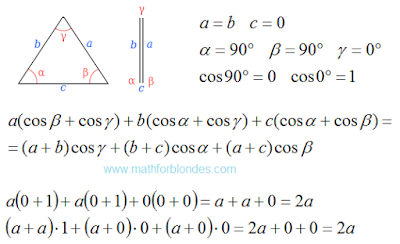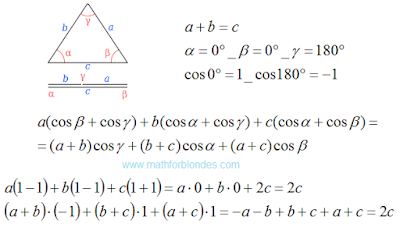## 3/28/2016

### Degenerate triangle

Finally, we will check the law of cosines to the perimeter in the degenerate triangle. There can be two options. If we decrease the base of an isosceles triangle to zero, we obtain the two overlapping segments. The sum of the angles of this degenerate triangle is 180 degrees.Degenerate triangle

If we combine the upper vertex of the triangle with the base, we get the second type of degenerate triangles. This segment equal to the sum of the other two segments. The sum of the angles are also equal to 180 degrees.Degenerate triangle

A degenerate triangle - is the lower limit of the application of the law of cosines. After that we will look at the law of cosines in general.